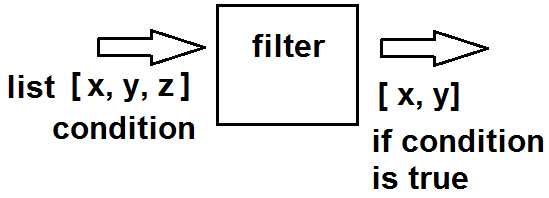﻿ How to Create a Filter Function in Python ﻿# How to Create a Filter Function in PythonIn this article, we go over how to create a filter function in Python

Before we do that, let's first go over what a filter function is?

A filter function is basically a function that filters out undesired results from a list.

It's a very easy concept to understand.

Let's say that we have a list of numbers and we only want numbers greater than 50 to be returned in the new filtered list.

This condition would use a filter function in Python.

The following diagram below helps to illustrate the filter function in Python.As you can see from the diagram, a list is passed into this filter function. The filter function has a condition that it tests for in each element (e.g, if the number is greater than 50). The modified list (with the condition) is then returned as output. Only those elements that meet the condition are part of the new modified list.

So into a filter() function in Python, you pass in 2 parameters, just like with a map() function. The first parameter is the function (the operation to carry out) and the second parameter is the list (or iterable object).

So this is a filter function in Python.

In the following code shown below, we create a filter() function that only returns elements in a list that are greater than 50.

So first we create a list. This list is composed of 5 numbers: 100,75,24,20,55

We then create a filter() function and assign it to the over50 variable.

Inside of this filter() function, the function we pass in is a lambda Therefore, we don't have to create some external function. We create a lambda function on the spot. We then pass in the second parameter, which is our iterable object, the list.

In order to print out the resultant modified list, we have to print out the filter function typed casted into a list.

We then get as output, [100, 75, 55]

You may ask how the filter() function is different than the map() function, for instance?

What if we had simply used the map() function instead of the filter() function?

The problem is, the map() function would return a list of boolean values of True and False instead of the actual values of the list. If I had run this same function with a map() function instead of a filter() function, the output would have been, [True, True, False, False, True]

A filter() function returns the actual values that meet the criteria. So this is why filter() functions are used when we want to return values that only meet a certain condition.

And this is how to use filter functions in Python.

Related Resources

How to Randomly Select From or Shuffle a List in Python